## Case Study: Reverse Ordering Strings

Provided we just settle for the UTF16 ranking, the most efficient way to sort a string array in reverse order is, `myStrings.sort().reverse()`. This code first applies the default sort routine then it simply reverses the array. Two native functions are invoked, no callback is in use, so that's the quickest solution.

In second place, one could use a custom `revCompare` function looking like `function(x,y){ return y < x ? -1 : +(y != x) }`. It still provides acceptable performance but is on average three to four times slower than the previous strategy (tested on 1,000 items.)

The worst solution—although inventive—would be to apply a bitwise negation to all UTF16 codes before calling the default `array.sort()`, then restore the original items. The underlying idea is to convert existing elements into something that supports the native sort (circumventing the injection of a callback function.) Unfortunately, this trick would only work if the conversion-restoration time was negligible compared to the time saved in applying a native sort.

Taking `myStrings.sort().reverse()` as the reference routine, the bit-inversion approach is from 3X to 50X slower (!) depending on both array's size and individual string length. There is definitely no point in using this strategy in common projects, as shown below.

```// =====================================
// Sorting strings in reverse order - Benchmark
// =====================================

const ARR_SIZE = 1e3,  // test: 10,100,1e3,1e4
STR_SIZE = 5,    // test: 1..10
LOOP = 20;       // increase LOOP to refine average time.

// Inject random strings (length=sz) in the given array.
// ---
const feedRandomStrings = function(a,sz,  chr,i,s)
{
chr = String.fromCharCode;
i = a.length;
while( i-- )
{
for( s='' ; s.length < sz ;
s += chr(0x41 + 58*Math.random())
);
a[i] = s;
}
return a;
};

// Perform a 'Binary Inversion' on string elements.
// ---
const binInversion = function(a,  chr,i,s,n,r,j)
{
chr = String.fromCharCode;
i = a.length;
while( i-- )
{
n = (s=a[i]).length;
for( r='', j=-1 ; ++j < n ; r += chr(~s.charCodeAt(j)) );
a[i] = r;
}
return a;
};

const revCompare = function(x,y){ return y < x ? -1 : +(y != x) };

var Benchmark = {
"Native Sort and Reverse" : function F(a)
{
// Simply call sort() and reverse().
// ---
F.data = a.concat();
\$.hiresTimer;
F.data.sort().reverse();
F.timer = \$.hiresTimer;
},
"Custom Compare Function" : function F(a)
{
// Reverse order based on a custom
// compare function.
// ---
F.data = a.concat();
\$.hiresTimer;
F.data.sort(revCompare);
F.timer = \$.hiresTimer;
},
"Using Binary Inversion" : function F(a)
{
// Reverse order based on bit inversion
// before and after a native sort.
// ---
F.data = a.concat();
\$.hiresTimer;
binInversion(F.data);
F.data.sort();
binInversion(F.data);
F.timer = \$.hiresTimer;
},
};

// Start the tests.
// ---
var myStrings = new Array(ARR_SIZE),
results = [],
tmRef = 0,
k, f, eq, i, t;

i = LOOP;
while( i-- )
{
feedRandomStrings(myStrings, STR_SIZE);
eq = 0;
for( k in Benchmark )
{
if( !Benchmark.hasOwnProperty(k) ) continue;

(f=Benchmark[k])(myStrings);

results[k] = f.timer + (results[k]||0);

if( !eq )
{
eq = f.data.toSource();
continue;
}

if( eq != f.data.toSource() )
{
throw new Error("Equality check failed for: " + k);
}
}
}

// Show the results.
// ---
results.push(localize(
"ARRAY SIZE: %1 - STRING SIZE: %2\r\r"
,ARR_SIZE
,STR_SIZE
));

for( k in Benchmark )
{
if( !Benchmark.hasOwnProperty(k) ) continue;

results.push(localize(
"%1 ( %2 \xB5s )"
,k
,t=Math.round(results[k]/LOOP)
));

if( !tmRef )
{
tmRef = t;
results.push("Time ratio: 1 [ref.]\r");
}
else
{
results.push(localize(
"Time ratio: %1 - Equality check: OK\r"
, (t/tmRef).toPrecision(2)
));
}
}

```

No miracle occurs… except for single-character strings: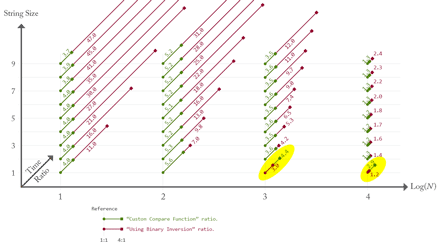Relative to the reference time, the ratio is very stable for the custom compare function—in green—whatever the string size and the array length. By contrast, binary inversion ratio—in red—substantially increases as the elements are getting longer. Let N be the number of items, S be the string size, then the total number of elementary bitwise operations is of `2×N×S` order. Noting that such value is on average similar to, or higher than `5×N×Log(N)`, and keeping in mind that `revCompare` only involves string comparisons while `binInversion` also includes a nested loop and string manipulations, it is easy to explain the slowness of our third strategy.

However, `2×N×S` becomes lower than `5×N×Log(N)` when very short strings or huge arrays are in use. This is why we see a dramatic effect at `N=1000` and `S=1` (single character). Here binary inversion is only 3X slower (relative to reference time), while custom compare is 4.4X slower. Thus, binary inversion is about 1.5X faster than custom compare in this special case. And the effect is even more pronounced for 10,000 elements.

I've studied the interesting case of `S=1` for `N` varying between 25 and 1,500. During this benchmark the useless inner loop of `binInversion` has been removed as follows:

```// Edited function, assuming that every
// string has a single character.
// ---
const binInversion = function(a,  chr,i)
{
chr = String.fromCharCode;
i = a.length;
while( i-- )
{
a[i] = chr(~a[i].charCodeAt(0));
}
return a;
};
```

We then observe that our not-so-weird routine starts to win as soon as N exceeds 50, and it becomes more and more efficient compared to the callback strategy: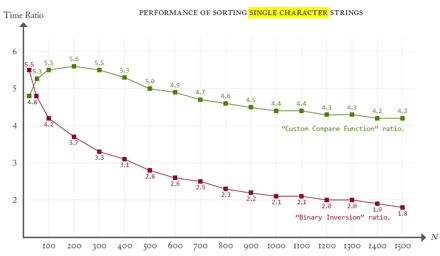The general rule we learn from this case study is: instead of using a custom compare function, converting data before and after a native sort can very appreciably speed up the job as long as the conversion routine runs in linear time and has a low factor relative to `5×Log(N)`.

Note. — In Part I we showed that a custom sort requires on average `5×N×Log(N)` elementary comparisons. Hence, a linear process that runs in `O(K×N)` has good chances of beating a custom sort if `K` is small compared to `5×Log(N)`. About the Big O notation, see Wikipedia's article.

## Refactoring the Model

Let's now introduce an abstract description of the task “sorting an array.” This involves four notions:

1. Items. — Items are the actual elements owned by the array. They can be of any type, including objects or arrays, so we shouldn't regard items as in essence comparable.

2. Keys. — Given an item, the first step is to extract, or to associate, a sort key, that is, a value that can be used for comparison. For example, if the array describes a set of employees (items), then we could choose their names, or their ages, as possible keys, depending on how we need to order them. Keys are usually scalar values (strings or numbers) but they do not determine a unique way of comparing. They only embody what is relevant for sorting.

3. Weights. — The process must then be able to associate a weight to any key. A weight is normally a number, or a sequence of numbers (string), that determines how keys are to be evaluated.

4. Comparing. — Comparing is the process of deciding whether two weights are equal, or which of the two is the lower (or higher) according to our sorting criteria. JavaScript expects a compare function to return either a negative number, a positive number, or zero, in a deterministic way.

In a perfect world, items would be keys and keys would be weights. This is exactly what happens when we call `myStrings.sort()`. No additional treatment is needed because the items (strings) are taken as sort keys and provide their own weight, that is, a sequence of UTF16 character codes which is smoothly compared to another sequence, thanks to the native routine.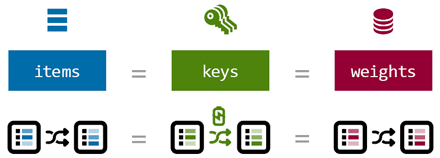Reminder. — The native sort routine is the one that instantly—or at least very efficiently—reorders an array of UTF16 sequences.

Things are very different in the worst case. The script needs to provide a custom callback routine which—inside a loglinear time stage—must both extract keys (from items), compute weights (from keys), and compare weights according to some specific convention. Even if calculations are optimized through cache systems (item-to-key and/or key-to-weight relationships), we have already noted how expensive and redundant are these operations: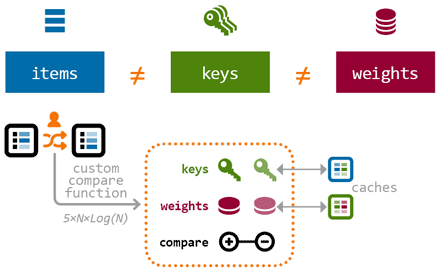The issue in the above pattern is, finding/managing keys and weights could be done in linear time. The only operation that actually requires a loglinear process is comparing weights.

Now, suppose the following conditions are satisfied:

C1. Given the original array `myArr`, we can build in linear time a proxy array `myProxy` collecting the weights of `myArr`'s associated keys.

C2. Weights in `myProxy` are fully encoded in UTF16 character sequences (i.e., strings.) This means that `myProxy` is an ideal-case array where items `=` keys `=` weights.

C3. Given an ordered array of weights—that is, `myProxy.sort()`—we can in linear time reorder `myArr` items in sync with weights.

Then we have another method for sorting `myArr` as needed, without using the custom compare pattern at all. C1 + C2 lead in linear time to a proxy array which supports the native sort, then C3 allows to transfer the induced order to the original array: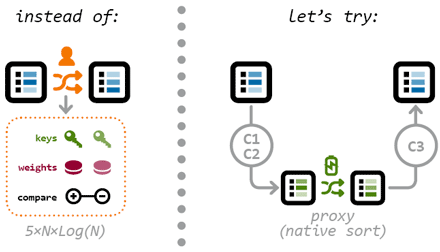This alternative strategy is attractive but has a serious counterpart: C3 is often hard to implement. First, there is no natural link between a weight in `myProxy` and the corresponding indices in `myArr`. Furthermore, reordering `myArr` according to some permutation requires a temporary copy of the original array, which increases the computation time.

But these issues can be solved using the following trick: appending to each weight the original item index (or even the original item itself, if it can be stringified) so that a permanent link is maintained between weights and items. This workaround will not affect weight ordering since characters that encode weights come before those which encode item data. One condition, however, is to know exactly how many characters are used for encoding the actual weight, in order to extract item data once `myProxy.sort()` is complete.

In the special case where items are string-like values, it's even possible to temporarily insert weights in `myArr`, run the native sort, then restore the original items:Anyway, if the data structure is more complex, you still have the option to use `uneval` and `eval` to manage data as strings. Here is a generic implementation of this idea:

```function sortByWeight(/*arr&*/a,/*fct*/toUtf16,/*uint*/weightSize)
{
var i, t;

// Convert a[i] into "<weight(i)><uneval(a[i])>",
// toUtf16 being a func that returns weight(i) as UTF16 sequence.
// ---
for( i=a.length ; i-- ; a[i] = toUtf16(t=a[i]) + uneval(t) );

// Native sort.
// ---
a.sort();

// Remove weights and restore item data.
// weightSize *must* be the length of any weight string.
// ---
for( i=a.length ; i-- ; a[i] = eval(a[i].substr(weightSize)) );
};

// ---
// SAMPLE USAGE
// ---

var myArr = [
//  name,  age
// -----------
[ "John",   38 ],
[ "Emma",   41 ],
[ "Bill",   28 ],
[ "Bob",    25 ],
[ "Sofia",  31 ]
];

// Sort items by increasing ages
// ---
sortByWeight(
myArr,
function(item){ return String.fromCharCode(item) },
1);

```

In summary, we have tools to completely avoid the use of a compare function. The whole question is to evaluate under which circumstances those tools are more efficient than ordinary methods. A basic flag might be defined: if your callback function spends more time in searching keys and/or computing weights, and less time in comparing them, there is good chances that linearizing these steps will be more effective.

## Application: Sorting Integers in the Range 0..65,535

Let `arr` be an array of positive integers such as each element remains in the interval `[0..65535]`. What could be more familiar than that?

The easiest way to sort those numbers in ascending order is, of course,
`arr.sort( function(x,y){ return x-y } )`. But this is not in general the best approach…

Indeed, any integer `i` such as `0 <= i <= 0xFFFF` can be encoded into a single UTF16 character using `String.fromCharCode(i)`. And any UTF16 character `c` can be converted back to the original integer using `c.charCodeAt(0)`. This is an ideal circumstance for testing our alternative method, although the compare function (`return x-y`) sounds extremely simple and fast.

Here is the alternative stuff:

```function sortIntegers(/*uint[]&*/arr,  i,chr)
// -------------------------------------
// arr must contain integers between 0 and 65535 (0xFFFF).
{
// Encode into UTF16 weights.
// ---
chr = String.fromCharCode;
for( i=arr.length ; i-- ; arr[i] = chr(arr[i]) );

// Native sort.
// ---
arr.sort();

// Restore original numbers.
for( i=arr.length ; i-- ; arr[i] = arr[i].charCodeAt(0) );
}

// Test
// ---
var arr = [65000,123,459,0,5431,777,23999];

sortIntegers(arr);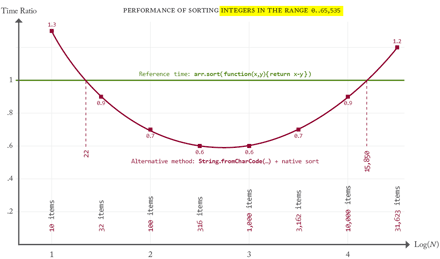As you can see, the (alter)native sort runs on average `1/0.6 = 1.67` times faster when the array size is between 316 and 1,000—which is a very common case. More generally, the alternative method remains the best for any array size varying from about 30 to 15,800 items!